# Posterior Distribution of μ and σ for Normal Distribution Based on Unknown Prior

### Home | Academic Articles

The basics

A normal distribution has two parameters, the mean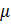which indicates where the bell curve is centered and the standard deviation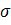which indicates the shape of the bell curve. From a frequentist point of view,andare fixed quantities. From a Bayesian point of view, they are random variables each with their own distribution, mean and standard deviation.

If a researcher has a set of data that appears to follow a normal distribution, we would like to find the distributions ofand.

If the researcher does not have a clear idea as to what distributionandjointly follow, Jeffreys suggested a diffuse prior distribution to indicate this lack of knowledge. When this prior distribution is combined with the data (known as the likelihood), the joint posterior distribution ofanddoes not follow any readily identifiable distribution.

However, once we solve for justfrom the joint posterior distribution, we find that it follows a t distribution with the mean equal to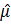which represents the sample mean of the data and the variance equal to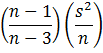in which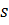represents the sample standard deviation of the data. Note that the sample size needs to be more than 3 in order to have a standard deviation. The other thing to note is that as the sample size increases, the standard deviation ofgets closer to zero.

Similarly, once we solve for justfrom the joint posterior distribution, we find that it follows an inverse gamma distribution with the mean equal to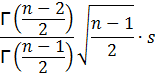where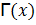is the gamma function of x. To have a mean, the sample size needs to be more than 1. The variance is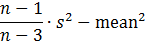To derive the standard deviation, we take the square root of the above quantity. To have a standard deviation, the sample size needs to be more than 3.

Example

Suppose a researcher takes a sample of 10 observations of people buying gas at a service station:

 39.62 48.21 52.48 57.06 57.24 60.04 63.64 68.05 73.98 81.24

Analysis indicates the data is normally distributed.

We have= 60.16,= 12.2545,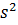= 150.17 and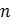= 10.

The posterior distribution ofindicates it follows a t distribution with a mean of 60.16 and variance of (9/7)(150.17/10) = 19.3078 or a standard deviation of 4.39 with 9 degrees of freedom.

Employing Chebyshev’s theorem, at least 8/9 of the distribution lies between 60.16 – 3(4.39) = 46.99 and 60.16 + 3(4.39) = 73.33.

The posterior distribution offollows an inverse gamma distribution with a mean of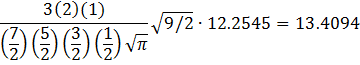The variance is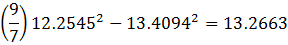The standard deviation is then the square root of 13.2663 which is 3.64.

Employing Chebyshev’s theorem, at least 8/9 of the distribution lies between 13.41 – 3(3.64) = 2.49 and 13.41 + 3(3.64) = 24.33.

Then, returning to the distribution of X, we can construct a table indicating the range of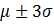depending on their values:

 μ | σ 2.49 13.41 24.33 46.99 (39.52, 54.46) (6.76,87.22) (0, 119.98) 60.16 (52.69, 67.63) (19.93, 100.39) (0, 133.15) 73.33 (65.86, 80.80) (33.10, 113.56) (0.34, 146.32)

Of these ranges, the one with μ = 60.16 and σ = 13.41 seems the most plausible. As more data is added, the range of μ and σ will tighten up. For example, I generated 1000 normal random numbers with a mean of 60.16 and standard deviation of 13.41. The mean of the data is 60.21 and the sample variance is 182.0687. Based on this data, μ follows a t distribution with a mean of 60.21 and standard deviation of 0.43 and σ follows an inverse gamma distribution with a mean of 13.50 and standard deviation of 0.30.

Technical details

If the researcher is starting from scratch, the joint prior distribution ofandshould convey this. The suggestion made by Jeffreys is to have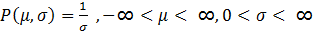.

Since our data appears to follow a normal distribution, each value y follows this distribution: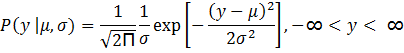Given the random sample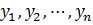, the likelihood function is: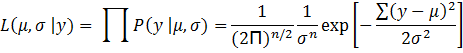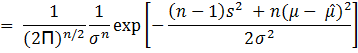whererepresents the sample mean of the data.

The expression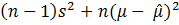is derived as follows: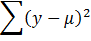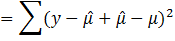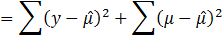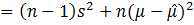The first term is derived from the fact that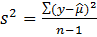.

There is no middle term from FOIL since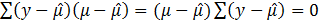.

Then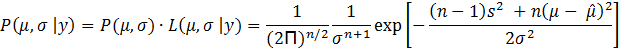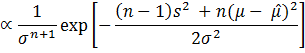Posterior Distribution of μ

To derive the posterior distribution of, we integrate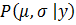with respect to.

We use the substitution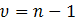in which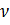represents the degrees of freedom. The result is: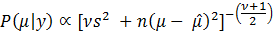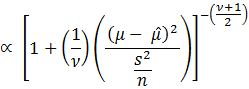Thus,follows a t distribution.

If we let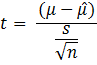then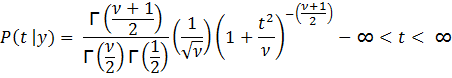whereis the gamma function of x.

To find the mean of t, E(t | y), we have: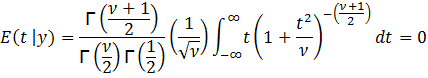This follows since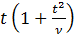is an odd function of t. From that we derive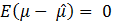and consequently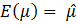provided> 1. Note that if= 1, the integral does not provide a finite solution. (In fact, t would follow a Cauchy distribution.)

Since E(t) = 0, the variance of t, Var(t) = E(t2)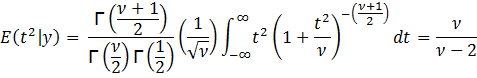Since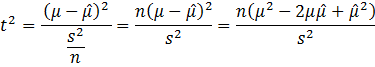then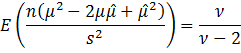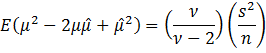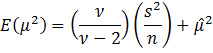This follow from the previous derivation of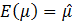.

Then,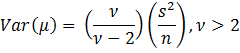This indicates that as the sample size increases, the variance ofdecreases.

Posterior Distribution of σ

To derive the posterior distribution of, we integratewith respect to.

We use the substitution. The result is: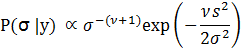This is in the form of an inverse Gamma distribution. Thus,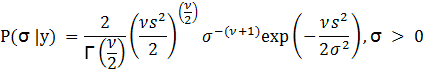In this case,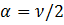and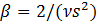.

To find the mean of σ, E(σ | y), we have: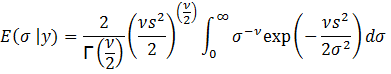Let y = 1/σ2. Then σ2 = y-1 leading to σ = y-0.5 and = -0.5y-1.5 dy. Substituting, we get: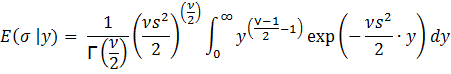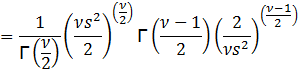The last line follows from the equation for the gamma function: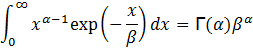Then,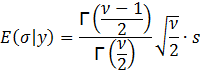To find the variance of σ, we need E(σ2 | y):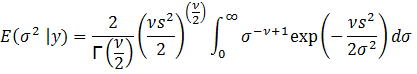Again, let y = 1/σ2. Then σ = y-0.5 and = -0.5y-1.5 dy. Substituting, we get: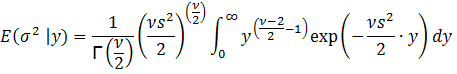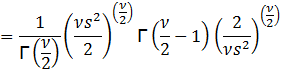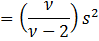Then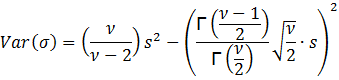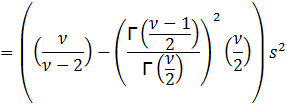Thus, we need> 2 in order to have a variance and subsequently a standard deviation.

Reference:

Zellner, Arnold. An Introduction to Bayesian Inference in Econometrics. New York: John Wiley & Sons, 1970.